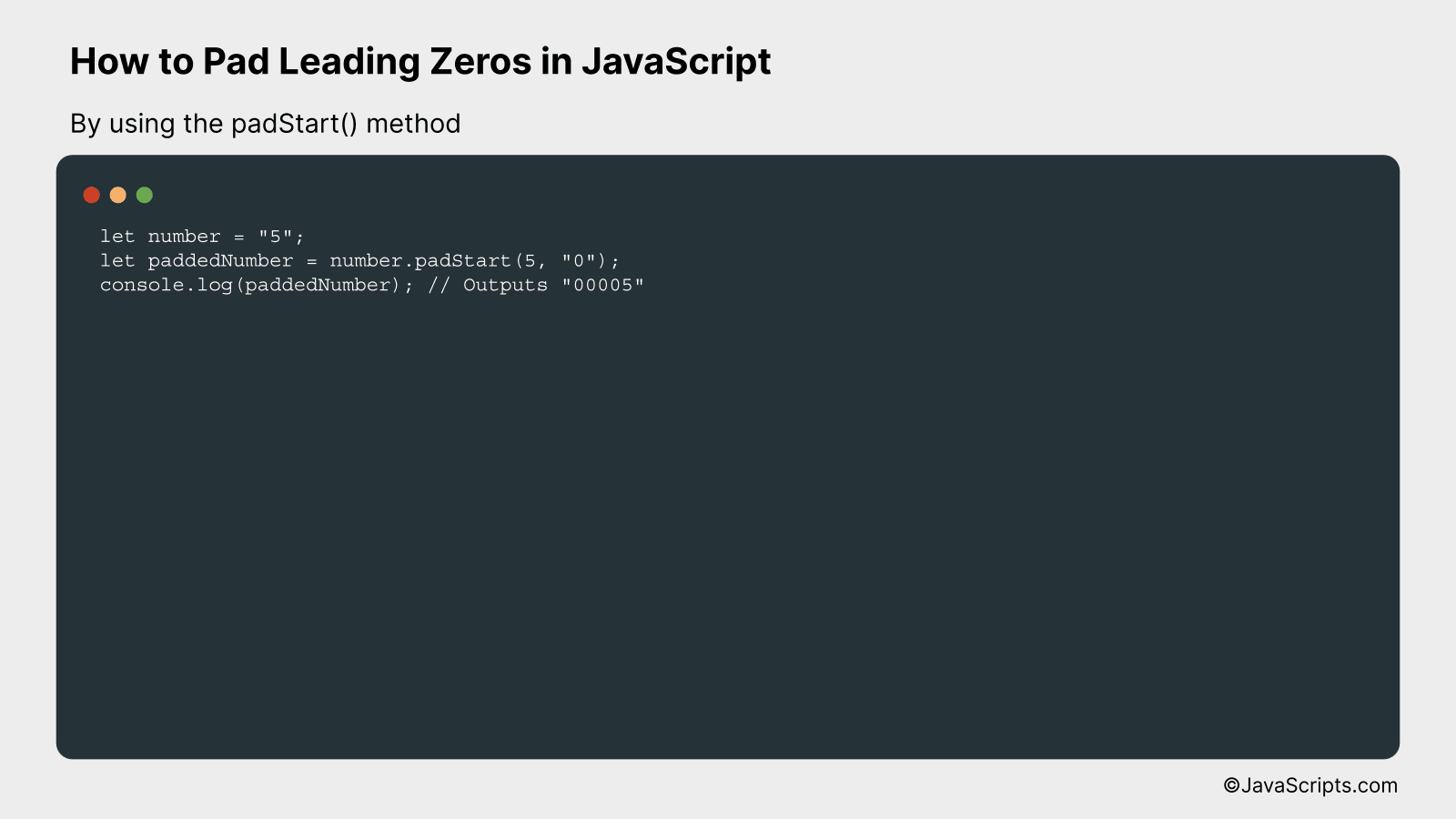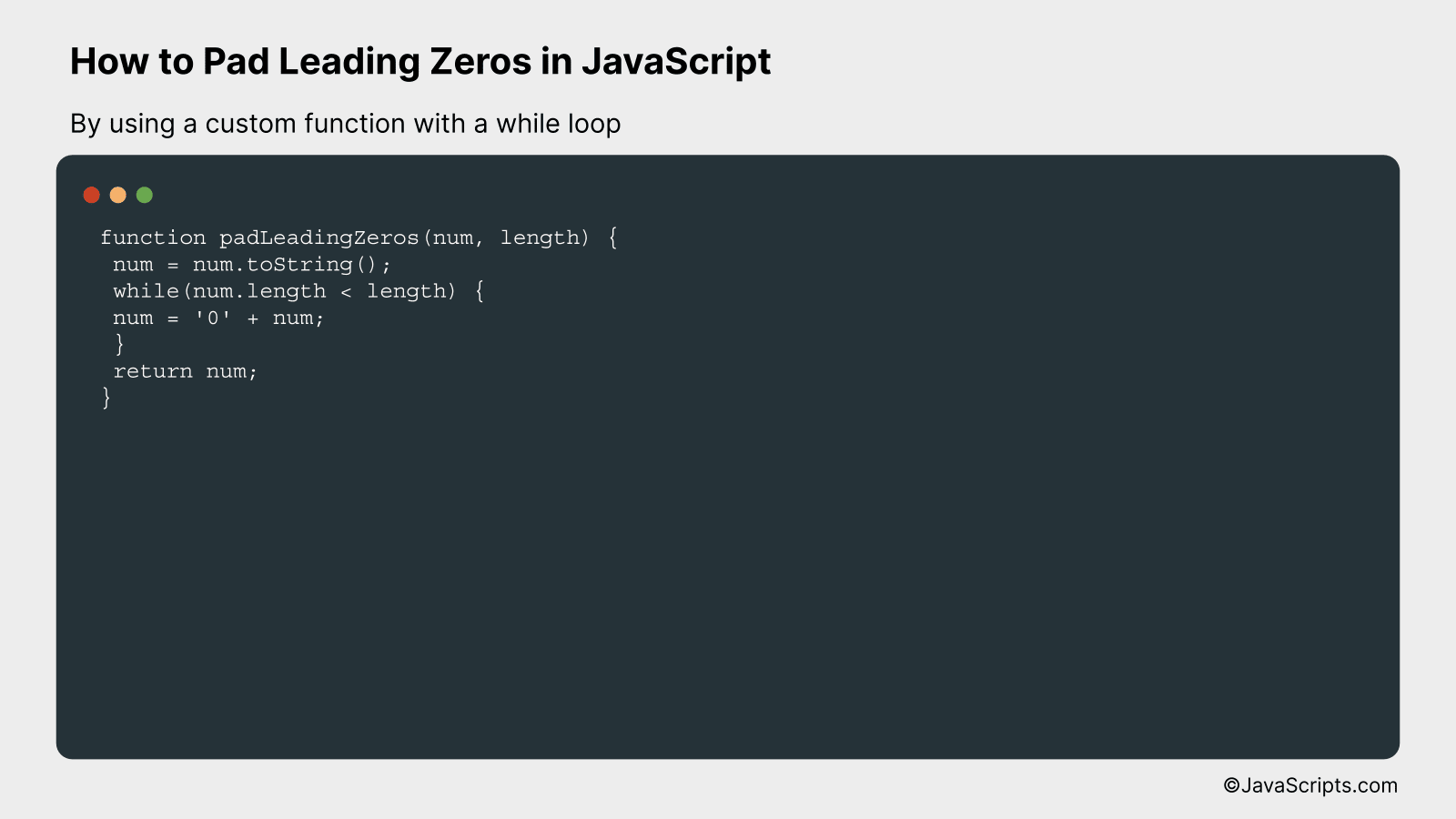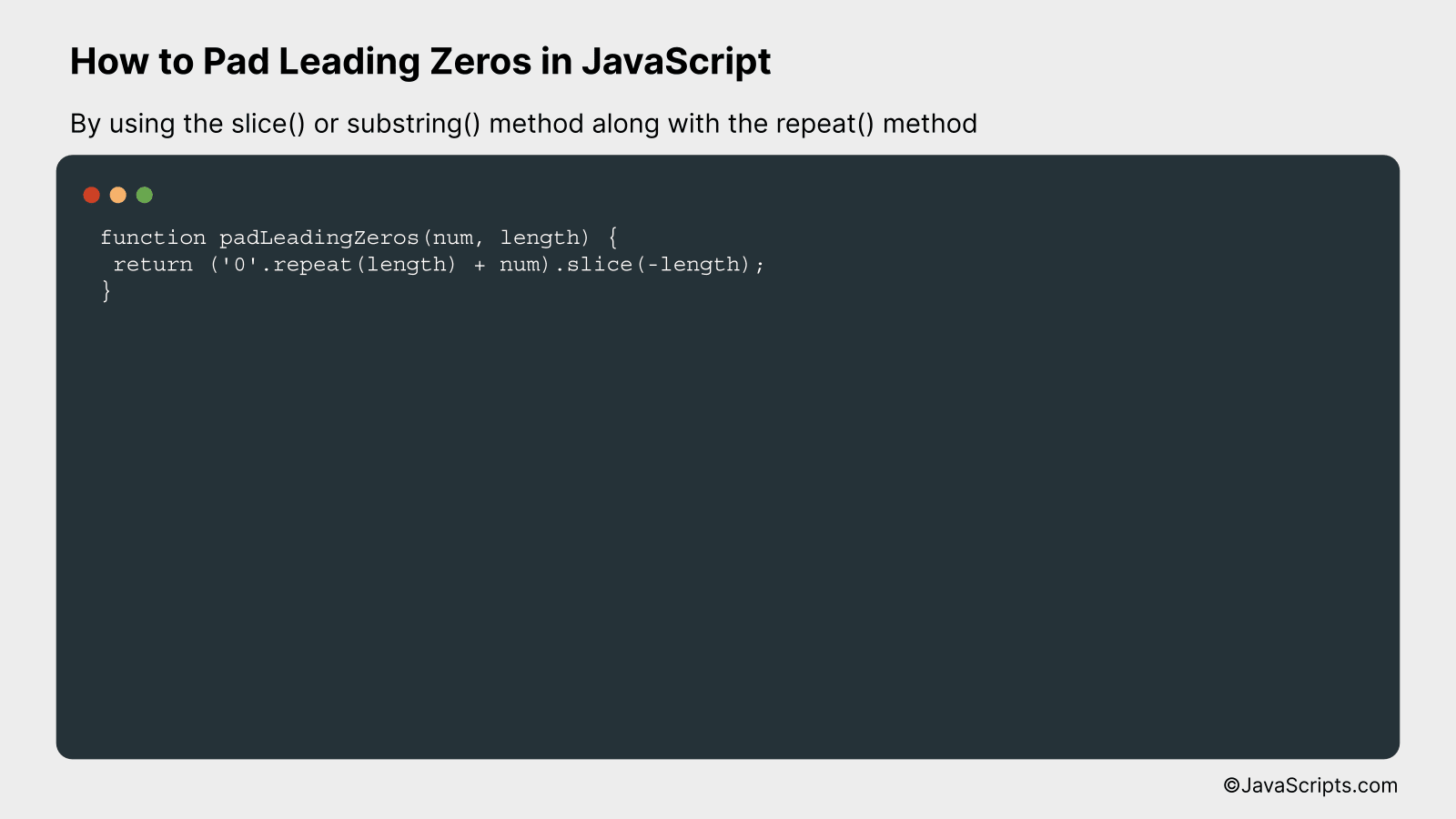Leading zeros: those silent placeholders that help to keep our numbers uniform and orderly. Often overlooked, they play a crucial role in formatting numbers, especially in the world of programming. You might wonder, how can we add these zeros at the start of a number? Well, JavaScript has a few tricks up its sleeve that can help us achieve this.

This task might seem daunting if you’re new to JavaScript, but don’t worry, it’s simpler than you think. We’re going to walk through step by step, using easy-to-understand language. By the end, you’ll be padding leading zeros like a pro!

In this post, we will be looking at the following 3 ways to pad leading zeros in JavaScript:

• By using the padStart() method
• By using a custom function with a while loop
• By using the slice() or substring() method along with the repeat() method

Let’s explore each…

## #1 – By using the padStart() methodThe padStart() method in JavaScript is a string function that pads the current string with a given string (repeated, if needed) until the resulting string reaches the provided length. The padding is applied from the start of the current string. Let’s understand this by considering an example where we want to pad a number with leading zeros.

``````
let number = "5";
``````

### How it works

The padStart() method pads the current string with another string (second argument) until the resulting string reaches the given length (first argument). The padding is applied from the start or beginning of the current string. In our example, we padded a string representation of a number with leading zeros until its length is 5.

• Initially, we declare a variable ‘number’ with a value of “5”.
• Then, we use the padStart() method on this string. The first argument is the total length we desire for our string, which is 5 in this case.
• The second argument is the string with which we want to pad our initial string. We use “0” here to add leading zeros.
• The padStart() method therefore adds enough zeros to the beginning of our ‘number’ string to make its length equal to 5. If ‘number’ was already 5 characters long or longer, no padding would occur.
• Finally, we log the result to the console, and we see that our number string is now “00005”, padded with zeros to reach a length of 5 characters.

## #2 – By using a custom function with a while loopThis is a custom JavaScript function that pads leading zeros to a number up to a specified length using a while loop. For example, if we want to pad the number 7 to a length of 3, we get “007”.

``````
num = num.toString();
while(num.length < length) {
num = '0' + num;
}
return num;
}
```
```

### How it works

This function first converts the number to a string. Then, it appends a '0' to the front of the string until it reaches the desired length. The padded string is then returned.

• The function takes two inputs: 'num', the number to be padded, and 'length', the desired length of the string after padding.
• The number 'num' is first converted into a string.
• The function enters a while loop that continues as long as the length of the string is less than the desired length.
• Inside the loop, a '0' is prepended to the start of the string.
• This process is repeated until the string reaches the specified length, effectively padding the number with leading zeros.
• The padded string is finally returned by the function.

## #3 - By using the slice() or substring() method along with the repeat() methodThis approach involves creating a string of zeros using the repeat() method, afterwards adding it to the beginning of the input number, and finally using the slice() or substring() method to return the desired length of characters from the end of the string.

For instance, if we need to pad the number '5' to a length of 5, we will first create a string '00000'. Then, we concatenate '00000' with '5' to get '000005'. Finally, we use the slice() method to return the last 5 characters: '00005'.

``````
return ('0'.repeat(length) + num).slice(-length);
}
``````

### How it works

The padLeadingZeros method takes two arguments: the number to be padded (num) and the desired length of the resulting string (length). It first creates a string of zeros equal to the desired length, then adds the number to the end of this string. Finally, it uses the slice() method to return the desired length from the end of the string.

• Repeat '0' for the desired length: '0'.repeat(length) generates a string of zeros.
• Concatenation: ('0'.repeat(length) + num) joins the number to the end of the zeros string.
• Slice: .slice(-length) returns the last 'length' characters from the string, effectively discarding any excess zeros from the start of the string.

Related:

In summary, padding leading zeros in JavaScript is a simple procedure that can be carried out with methods like 'padStart'. Such methods help format numbers and strings with ease, making your code cleaner and more efficient.

Understanding and applying these methods can take your JavaScript coding to the next level. Therefore, never stop learning and experimenting with these useful techniques to enhance your programming skills.